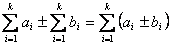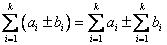## Can I expand or regroup the sum?

Yes, you can expand or regroup two sums as long as the numbers of elements are the same. Here are the formulas(Regrouping)(Expanding)

Notice that the total members between two sums are the same

Example< Previous | Next | Contents >